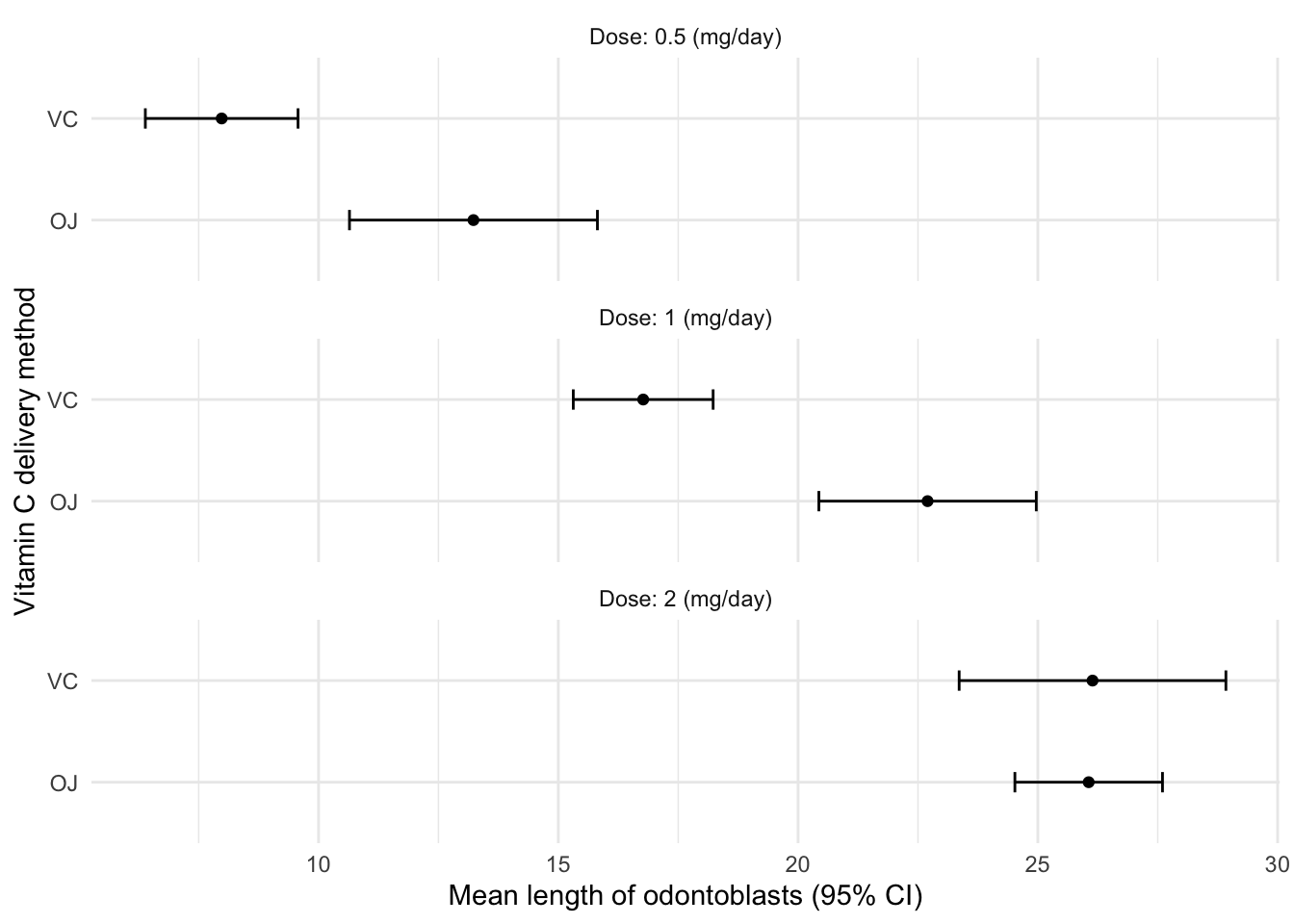# summary_se()

I’ve found myself on Winston Chang’s cookbook-r website a number of times before. My most recent visit was to refresh my memory on the strategy for plotting means with error bars with `ggplot2`:

http://www.cookbook-r.com/Graphs/Plotting_means_and_error_bars_(ggplot2)

The recipe makes use of `summarySE()` a function that is described in detail in another post that details strategies for summarizing data.

`summarySE()` is a custom function that computes the mean, standard deviation, count, standard error, confidence interval for a variable (“measurevar”) within defined grouping variables (“groupvars”).

Below is the code for the function, along with a working example of how to use it with the built-in “ToothGrowth” dataset (`?ToothGrowth`).

``````## Summarizes data.
## Gives count, mean, standard deviation, standard error of the mean, and confidence interval (default 95%).
##   data: a data frame.
##   measurevar: the name of a column that contains the variable to be summarized
##   groupvars: a vector containing names of columns that contain grouping variables
##   na.rm: a boolean that indicates whether to ignore NA's
##   conf.interval: the percent range of the confidence interval (default is 95%)
summarySE <- function(data=NULL, measurevar, groupvars=NULL, na.rm=FALSE,
conf.interval=.95, .drop=TRUE) {
library(plyr)

# New version of length which can handle NA's: if na.rm==T, don't count them
length2 <- function (x, na.rm=FALSE) {
if (na.rm) sum(!is.na(x))
else       length(x)
}

# This does the summary. For each group's data frame, return a vector with
# N, mean, and sd
datac <- ddply(data, groupvars, .drop=.drop,
.fun = function(xx, col) {
c(N    = length2(xx[[col]], na.rm=na.rm),
mean = mean   (xx[[col]], na.rm=na.rm),
sd   = sd     (xx[[col]], na.rm=na.rm)
)
},
measurevar
)

# Rename the "mean" column
datac <- rename(datac, c("mean" = measurevar))

datac\$se <- datac\$sd / sqrt(datac\$N)  # Calculate standard error of the mean

# Confidence interval multiplier for standard error
# Calculate t-statistic for confidence interval:
# e.g., if conf.interval is .95, use .975 (above/below), and use df=N-1
ciMult <- qt(conf.interval/2 + .5, datac\$N-1)
datac\$ci <- datac\$se * ciMult

return(datac)
}``````
``head(ToothGrowth)``
``````##    len supp dose
## 1  4.2   VC  0.5
## 2 11.5   VC  0.5
## 3  7.3   VC  0.5
## 4  5.8   VC  0.5
## 5  6.4   VC  0.5
## 6 10.0   VC  0.5``````
``````summarySE(ToothGrowth,
measurevar="len",
groupvars=c("supp","dose"),
conf.interval = 0.9)``````
``````##   supp dose  N   len       sd        se       ci
## 1   OJ  0.5 10 13.23 4.459709 1.4102837 2.585209
## 2   OJ  1.0 10 22.70 3.910953 1.2367520 2.267106
## 3   OJ  2.0 10 26.06 2.655058 0.8396031 1.539087
## 4   VC  0.5 10  7.98 2.746634 0.8685620 1.592172
## 5   VC  1.0 10 16.77 2.515309 0.7954104 1.458077
## 6   VC  2.0 10 26.14 4.797731 1.5171757 2.781154``````

### `tidyeval` approach

The cookbook-r site includes several solutions to the “Summary SE” problem. The `summarySE()` function (above) works well, and includes comments explaining each parameter. However, given my inexperience with `plyr` I find the code a little hard to understand … or at least harder than if it were written with `dplyr`. I decided to try to translate `summarySE()` into a syntax that I could use in a pipeline with `%>%`. To do so, I needed to learn a little about the `tidyeval` framework and its programming paradigm.

The code for the new function (`summary_se()`) is below, along with the same ToothGrowth example as above.

A few notes from my exploration:

• `enquo()` captures the bare variable name, and `!!` in a subsequent `dplyr` call will reference that variable
• `enquos()` and `!!!` work in a similar matter but can capture multiple variable names passed in via `...`
• It’s good practice to prefix argument names with `.` when writing functions that use `tidyeval` … the reason being that it is not likely that a user would have an existing column name that starts with `.`
``````summary_se <- function(.data, measure_var, ..., .ci = 0.95, na.rm = FALSE) {

measure_var <- dplyr::enquo(measure_var)
group_var <- dplyr::enquos(...)

.data %>%
group_by(!!! group_var) %>%
summarise(mean = mean(!! measure_var, na.rm = na.rm),
sd = sd(!! measure_var, na.rm = na.rm),
n = n(),
se = sd/sqrt(n),
ci = se * qt(.ci/2 + 0.5, n-1)) %>%
ungroup()

}``````
``````library(dplyr)
library(ggplot2)

ToothGrowth %>%
summary_se(len, supp, dose, .ci = 0.9)``````
``````## # A tibble: 6 x 7
##   supp   dose  mean    sd     n    se    ci
##   <fct> <dbl> <dbl> <dbl> <int> <dbl> <dbl>
## 1 OJ      0.5 13.2   4.46    10 1.41   2.59
## 2 OJ      1   22.7   3.91    10 1.24   2.27
## 3 OJ      2   26.1   2.66    10 0.840  1.54
## 4 VC      0.5  7.98  2.75    10 0.869  1.59
## 5 VC      1   16.8   2.52    10 0.795  1.46
## 6 VC      2   26.1   4.80    10 1.52   2.78``````
``````ToothGrowth %>%
summary_se(len, supp, dose, .ci = 0.9) %>%
mutate(dose = paste0("Dose: ", dose, " (mg/day)")) %>%
ggplot(aes(supp,mean)) +
geom_point() +
geom_errorbar(aes(ymin = mean - ci,
ymax = mean + ci),
width = 0.2) +
labs(x = "Vitamin C delivery method", y = "Mean length of odontoblasts (95% CI)") +
coord_flip() +
facet_wrap(~ dose, ncol = 1) +
theme_minimal()``````